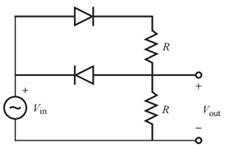Create an Account

Home / Questions / For the following circuit assuming ideal diodes and given

For the following circuit assuming ideal diodes and given

For the following circuit, assuming ideal diodes and given R = 1 kΩ and Vin = 10 sin( πt ) V, plot the output voltage Vout on axes with labeled scales for two periods of the input.Jul 27 2020 View more View LessSubscribe To Get Solution